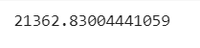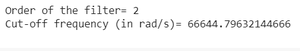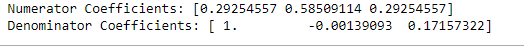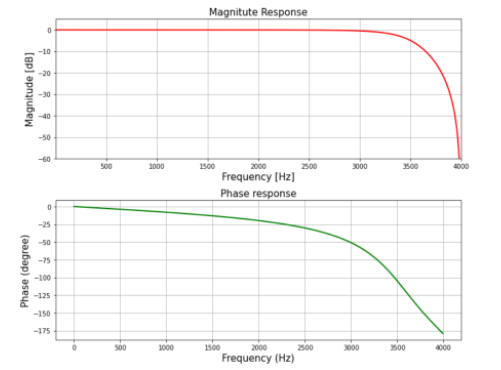# Design IIR Lowpass Butterworth Filter using Bilinear Transformation Method in Scipy- Python

• Last Updated : 10 Nov, 2021

IIR stands for Infinite Impulse Response, It is one of the striking features of many linear-time invariant systems that are distinguished by having an impulse response h(t)/h(n) which does not become zero after some point but instead continues infinitely.

## What is IIR Lowpass Butterworth ?

It basically behaves just like an ordinary digital Lowpass Butterworth Filter with an infinite impulse response.

Attention geek! Strengthen your foundations with the Python Programming Foundation Course and learn the basics.

To begin with, your interview preparations Enhance your Data Structures concepts with the Python DS Course. And to begin with your Machine Learning Journey, join the Machine Learning - Basic Level Course

The specifications are as follows:

• Sampling rate of 8 kHz
• Order of Filter 2
• Cutoff-frequency 3400Hz

We will plot the magnitude & phase response of the filter.

Step-by-step Approach:

Step 1: Importing all the necessary libraries.

## Python3

 `# import required library``import` `numpy as np``import` `scipy.signal as signal``import` `matplotlib.pyplot as plt`

Step 2: Define variables with the given specifications of the filter.

## Python3

 `# Given specification``N ``=` `2`  `# Order of the filter``Fs ``=` `8000`  `# Sampling frequency in Hz``fc ``=` `3400`  `# Cut-off frequency in Hz` `# Compute Design Sampling parameter``Td ``=` `1``/``Fs`

Step 3: Computing the cut-off frequency

## Python3

 `# Compute cut-off frequency in radian/sec``wd ``=` `2``*``np.pi``*``fc``print``(wd)  ``# Cut-off frequency in radian/sec`

Output:Step 4: Pre-wrapping the analog frequency

## Python3

 `# Prewarp the analog frequency` `wc ``=` `(``2``/``Td)``*``np.tan(wd``*``Td``/``2``)``print``(``'Order of the filter='``, N)  ``# Order` `# Prewarped analog cut-off frequency``print``(``'Cut-off frequency (in rad/s)='``, wc)`

Output:Step 5: Designing the filter using signal.butter() function and then performing bilinear transformation using signal.bilinear() function

## Python3

 `# Design analog Butterworth filter using signal.butter function` `b, a ``=` `signal.butter(N, wc, ``'low'``, analog``=``'True'``)``# Perform bilinear Transformation` `z, p ``=` `signal.bilinear(b, a, fs``=``Fs)` `# Print numerator and denomerator coefficients of the filter``print``(``'Numerator Coefficients:'``, z)``print``(``'Denominator Coefficients:'``, p)`

Output:Step 6: Computing the frequency response of the filter using signal.freqz() function and plotting the magnitude and phase response

## Python3

 `# Compute frequency response of the filter using signal.freqz function``wz, hz ``=` `signal.freqz(z, p, ``512``)` `# Plot filter magnitude and phase responses using subplot.``# Convert digital frequency wz into analog frequency in Hz``fig ``=` `plt.figure(figsize``=``(``12``, ``10``))` `# Calculate Magnitude from hz in dB``Mag ``=` `20``*``np.log10(``abs``(hz))` `# Calculate frequency in Hz from wz``Freq ``=` `wz``*``Fs``/``(``2``*``np.pi)` `# Plot Magnitude response``sub1 ``=` `plt.subplot(``2``, ``1``, ``1``)``sub1.plot(Freq, Mag, ``'r'``, linewidth``=``2``)``sub1.axis([``1``, Fs``/``2``, ``-``60``, ``5``])``sub1.set_title(``'Magnitude Response'``, fontsize``=``15``)``sub1.set_xlabel(``'Frequency [Hz]'``, fontsize``=``15``)``sub1.set_ylabel(``'Magnitude [dB]'``, fontsize``=``15``)``sub1.grid()`  `# Plot phase angle``sub2 ``=` `plt.subplot(``2``, ``1``, ``2``)` `# Calculate phace angle in degree from hz``Phase ``=` `np.unwrap(np.angle(hz))``*``180``/``np.pi``sub2.plot(Freq, Phase, ``'g'``, linewidth``=``2``)``sub2.set_ylabel(``'Phase (degree)'``, fontsize``=``15``)``sub2.set_xlabel(r``'Frequency (Hz)'``, fontsize``=``15``)``sub2.set_title(r``'Phase response'``, fontsize``=``15``)``sub2.grid()` `plt.subplots_adjust(hspace``=``0.5``)``fig.tight_layout()``plt.show()`

Output:Below is the implementation:

## Python3

 `# import required library``import` `numpy as np``import` `scipy.signal as signal``import` `matplotlib.pyplot as plt` `# Given specification``N ``=` `2`  `# Order of the filter``Fs ``=` `8000`  `# Sampling frequency in Hz``fc ``=` `3400`  `# Cut-off frequency in Hz` `# Compute Design Sampling parameter``Td ``=` `1``/``Fs` `# Compute cut-off frequency in radian/sec``wd ``=` `2``*``np.pi``*``fc``print``(wd)  ``# Cut-off frequency in radian/sec` `# Prewarp the analog frequency``wc ``=` `(``2``/``Td)``*``np.tan(wd``*``Td``/``2``)``print``(``'Order of the filter='``, N)  ``# Order` `# Prewarped analog cut-off frequency``print``(``'Cut-off frequency (in rad/s)='``, wc)` `# Design analog Butterworth filter using signal.butter function``b, a ``=` `signal.butter(N, wc, ``'low'``, analog``=``'True'``)` `# Perform bilinear Transformation``z, p ``=` `signal.bilinear(b, a, fs``=``Fs)` `# Print numerator and denomerator coefficients of the filter``print``(``'Numerator Coefficients:'``, z)``print``(``'Denominator Coefficients:'``, p)` `# Compute frequency response of the filter using signal.freqz function``wz, hz ``=` `signal.freqz(z, p, ``512``)` `# Plot filter magnitude and phase responses using subplot.``#Convert digital frequency wz into analog frequency in Hz``fig ``=` `plt.figure(figsize``=``(``10``, ``8``))` `# Calculate Magnitude from hz in dB``Mag ``=` `20``*``np.log10(``abs``(hz))` `# Calculate frequency in Hz from wz``Freq ``=` `wz``*``Fs``/``(``2``*``np.pi)` `# Plot Magnitude response``sub1 ``=` `plt.subplot(``2``, ``1``, ``1``)``sub1.plot(Freq, Mag, ``'r'``, linewidth``=``2``)``sub1.axis([``1``, Fs``/``2``, ``-``60``, ``5``])``sub1.set_title(``'Magnitude Response'``, fontsize``=``15``)``sub1.set_xlabel(``'Frequency [Hz]'``, fontsize``=``15``)``sub1.set_ylabel(``'Magnitude [dB]'``, fontsize``=``15``)``sub1.grid()` `# Plot phase angle``sub2 ``=` `plt.subplot(``2``, ``1``, ``2``)` `# Calculate phace angle in degree from hz``Phase ``=` `np.unwrap(np.angle(hz))``*``180``/``np.pi``sub2.plot(Freq, Phase, ``'g'``, linewidth``=``2``)``sub2.set_ylabel(``'Phase (degree)'``, fontsize``=``15``)``sub2.set_xlabel(r``'Frequency (Hz)'``, fontsize``=``15``)``sub2.set_title(r``'Phase response'``, fontsize``=``15``)``sub2.grid()` `plt.subplots_adjust(hspace``=``0.5``)``fig.tight_layout()``plt.show()`

Output:My Personal Notes arrow_drop_up